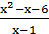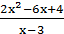### Sample Problem

The length of the base of a triangle in terms of x isand the height of the triangle is.

What is the area of the triangle in terms of x? Leave your answer in factored form.

Area: ___

#### Solution

A =× b × h

A =××=××= (x – 2)(x + 2)